基于TensorFlow.js的JavaScript机器学习

+关注继续查看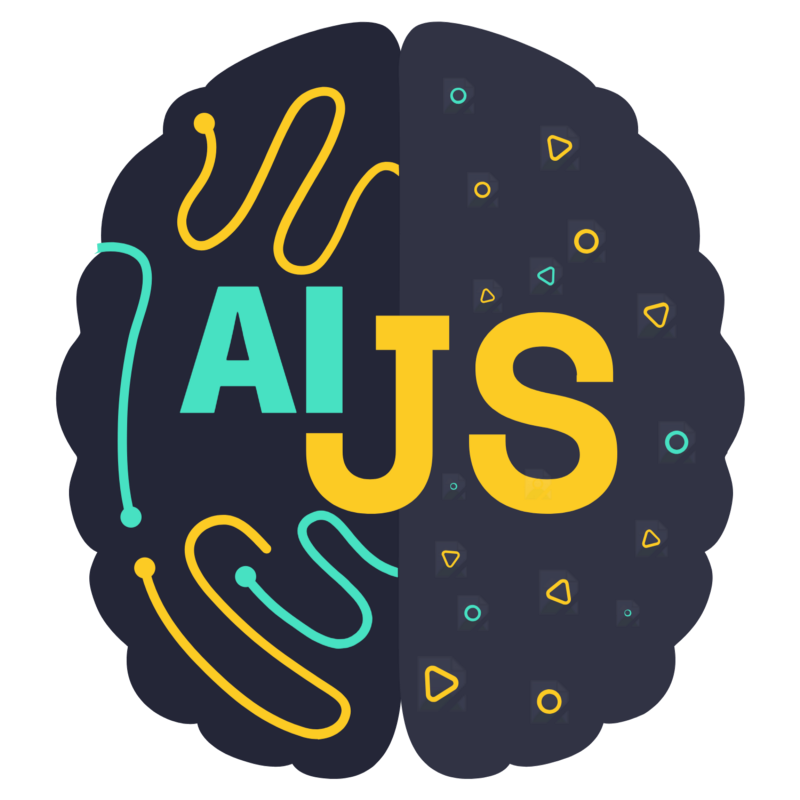Credits: aijs.rocks

2019年11个javascript机器学习库

Big.bitsrc.io

<!DOCTYPE html>
<html>
<title>TensorFlow.js Tutorial</title>
<!-- Import TensorFlow.js -->
<script src="https://cdn.jsdelivr.net/npm/@tensorflow/tfjs@1.0.0/dist/tf.min.js"></script>
<!-- Import tfjs-vis -->
<script src="https://cdn.jsdelivr.net/npm/@tensorflow/tfjs-vis@1.0.2/dist/tfjs-vis.umd.min.js"></script>
<!-- Import the main script file -->
<script src="script.js"></script>
<body>
</body>
</html>

console.log('Hello TensorFlow');

• tf是对tensorflow.js库的引用
• tfvis是对tfjs vis库的引用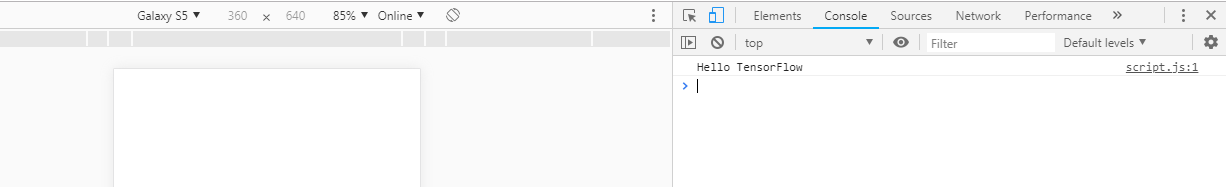Bit（GitHub上的Bit）是跨项目和应用程序共享可重用JavaScript代码的最快和最可扩展的方式。可以试一试，它是免费的：

Bit是开发人员共享组件和协作，共同构建令人惊叹的软件的地方。发现共享的组件…
Bit.dev

Ramda by Ramda·Bit

Bit.dev

async function getData() {
Const houseDataReq=await
fetch('https://raw.githubusercontent.com/meetnandu05/ml1/master/house.json');
const houseData = await houseDataReq.json();
const cleaned = houseData.map(house => ({
price: house.Price,
rooms: house.AvgAreaNumberofRooms,
}))
.filter(house => (house.price != null && house.rooms != null));

return cleaned;
}

async function run() {
// Load and plot the original input data that we are going to train on.
const data = await getData();
const values = data.map(d => ({
x: d.rooms,
y: d.price,
}));
tfvis.render.scatterplot(
{name: 'No.of rooms v Price'},
{values},
{
xLabel: 'No. of rooms',
yLabel: 'Price',
height: 300
}
);
// More code will be added below
}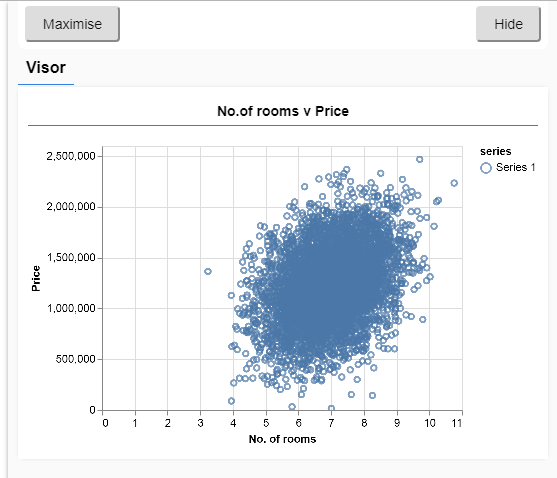function createModel() {
// Create a sequential model
const model = tf.sequential();

// Add a single hidden layer
model.add(tf.layers.dense({inputShape: , units: 1, useBias: true}));

return model;
}

const model = tf.sequential();

model.add(tf.layers.dense({inputShape: , units: 1, useBias: true}));

// Create the model
const model = createModel();
tfvis.show.modelSummary({name: 'Model Summary'}, model);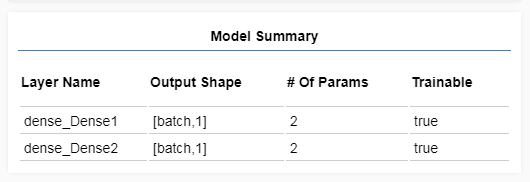function convertToTensor(data) {

return tf.tidy(() => {
// Step 1. Shuffle the data
tf.util.shuffle(data);
// Step 2. Convert data to Tensor
const inputs = data.map(d => d.rooms)
const labels = data.map(d => d.price);
const inputTensor = tf.tensor2d(inputs, [inputs.length, 1]);
const labelTensor = tf.tensor2d(labels, [labels.length, 1]);
//Step 3. Normalize the data to the range 0 - 1 using min-max scaling
const inputMax = inputTensor.max();
const inputMin = inputTensor.min();
const labelMax = labelTensor.max();
const labelMin = labelTensor.min();
const normalizedInputs = inputTensor.sub(inputMin).div(inputMax.sub(inputMin));
const normalizedLabels = labelTensor.sub(labelMin).div(labelMax.sub(labelMin));
return {
inputs: normalizedInputs,
labels: normalizedLabels,
// Return the min/max bounds so we can use them later.
inputMax,
inputMin,
labelMax,
labelMin,
}
});
}

// Step 1. Shuffle the data
tf.util.shuffle(data);

// Step 2. Convert data to Tensor
const inputs = data.map(d => d.rooms)
const labels = data.map(d => d.price);
const inputTensor = tf.tensor2d(inputs, [inputs.length, 1]);
const labelTensor = tf.tensor2d(labels, [labels.length, 1]);

//Step 3. Normalize the data to the range 0 - 1 using min-max scaling
const inputMax = inputTensor.max();
const inputMin = inputTensor.min();
const labelMax = labelTensor.max();
const labelMin = labelTensor.min();
const normalizedInputs = inputTensor.sub(inputMin).div(inputMax.sub(inputMin));
const normalizedLabels = labelTensor.sub(labelMin).div(labelMax.sub(labelMin));

return {
inputs: normalizedInputs,
labels: normalizedLabels,
// Return the min/max bounds so we can use them later.
inputMax,
inputMin,
labelMax,
labelMin,
}

async function trainModel(model, inputs, labels) {
// Prepare the model for training.
model.compile({
loss: tf.losses.meanSquaredError,
metrics: ['mse'],
});

const batchSize = 28;
const epochs = 50;

return await model.fit(inputs, labels, {
batchSize,
epochs,
shuffle: true,
callbacks: tfvis.show.fitCallbacks(
{ name: 'Training Performance' },
['loss', 'mse'],
{ height: 200, callbacks: ['onEpochEnd'] }
)
});
}

// Prepare the model for training.
model.compile({
loss: tf.losses.meanSquaredError,
metrics: ['mse'],
});

const batchSize = 28;
const epochs = 50;

batchSize指的是模型在每次运行迭代时将看到的数据子集的大小。常见的批量大小通常在32-512之间。对于所有问题来说，并没有一个真正理想的批量大小，描述各种批量大小的精确方式这一知识点本教程没有相关讲解，对这些有兴趣可以通过别的渠道进行了解学习。

epochs指的是模型将查看你提供的整个数据集的次数。在这里，我们通过数据集进行50次迭代。

return model.fit(inputs, labels, {
batchSize,
epochs,
callbacks: tfvis.show.fitCallbacks(
{ name: 'Training Performance' },
['loss', 'mse'],
{
height: 200,
callbacks: ['onEpochEnd']
}
)
});

model.fit是我们调用的启动循环的函数。它是一个异步函数，因此我们返回它给我们的特定值，以便调用者可以确定运行结束时间。

// Convert the data to a form we can use for training.
const tensorData = convertToTensor(data);
const {inputs, labels} = tensorData;

// Train the model
await trainModel(model, inputs, labels);
console.log('Done Training');

function testModel(model, inputData, normalizationData) {
const {inputMax, inputMin, labelMin, labelMax} = normalizationData;

// Generate predictions for a uniform range of numbers between 0 and 1;
// We un-normalize the data by doing the inverse of the min-max scaling
// that we did earlier.
const [xs, preds] = tf.tidy(() => {

const xs = tf.linspace(0, 1, 100);
const preds = model.predict(xs.reshape([100, 1]));

const unNormXs = xs
.mul(inputMax.sub(inputMin))

const unNormPreds = preds
.mul(labelMax.sub(labelMin))

// Un-normalize the data
return [unNormXs.dataSync(), unNormPreds.dataSync()];
});

const predictedPoints = Array.from(xs).map((val, i) => {
return {x: val, y: preds[i]}
});

const originalPoints = inputData.map(d => ({
x: d.rooms, y: d.price,
}));

tfvis.render.scatterplot(
{name: 'Model Predictions vs Original Data'},
{values: [originalPoints, predictedPoints], series: ['original', 'predicted']},
{
xLabel: 'No. of rooms',
yLabel: 'Price',
height: 300
}
);
}

const xs = tf.linspace(0, 1, 100);
const preds = model.predict(xs.reshape([100, 1]));

// Un-normalize the data
const unNormXs = xs
.mul(inputMax.sub(inputMin))

const unNormPreds = preds
.mul(labelMax.sub(labelMin))

return [unNormXs.dataSync(), unNormPreds.dataSync()];

.datasync（）是一种方法，我们可以使用它来获取存储在张量中的值的typedarray。这允许我们在常规的javascript中处理这些值。这是通常首选的.data（）方法的同步版本。

testModel(model, data, tensorData);1732 0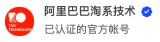33 0Java类加载器学习2——自定义类加载器和父类委托机制带来的问题
1102 05273 08月22日云栖精选夜读 | Java开发者必看！机器学习开发库精选！

2564 0[译] 写给机器学习工程师：如何测试Tensorflow模型
## 引言 这篇文章来自斯坦福大学计算与数学工程所（Institute for Computational & Mathematical Engineering）博士生Guillaume Genthial的[博客](https://guillaumegenthial.github.io/testing.html)。主要介绍了如何将工程界里已经得到充分认可的单元测试实践应用到算法建模的领域中，
1613 01050 0《构建实时机器学习系统》一1.6　实时应用对机器学习的要求

964 0【翻译】Sklearn与TensorFlow机器学习实用指南 —— 第16章 强化学习（下）
1611 01775 0
【方向】

707

5

《2021云上架构与运维峰会演讲合集》

《零基础CSS入门教程》

《零基础HTML入门教程》